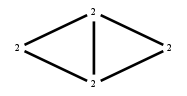# The Markov Bases Database

## G17g_bin

The binary graphical model of G17.

It is a hierarchical model of 4 variables. The dimension of the model is 11.The cardinality of the statespace is 16.

### Properties of the Markov basis

 Markov degree 2 4

 degree 2 4

The model has the following properties:

• All variables are binary.
• It is a graphical model.
• The minimal Markov basis is unique.
• The semigroup is normal.### FILES

 Markov basis: sufficient statistics matrix: G17g_bin.mar (201 b) G17g_bin.mat (534 b) G17g_bin.mod (29 b) G17g_bin.tar.gz (4.52 kb)